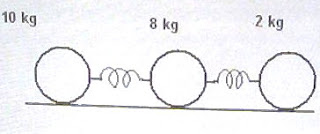## Pages

`“Life is like riding a bicycle.  To keep your balance you must keep moving.”–Albert Einstein`

## Thursday, October 8, 2009

### Multiple Choice Practice Questions on Rotation for AP Physics C

Some multiple choice practice questions (with solution) on rotational motion were posted earlier on this site. You can access those posts by clicking on the label ‘rotational motion’ or ‘rotation’ below this post. We will discuss a few more multiple choice questions (MCQ) for practice in this section today:

(1) A car moves forward on level road with uniform velocity of 4 ms–1. If there is no slipping of the tyres, the velocities (with respect to the road) of the points at the bottom, centre and the top of a tyre are respectively

(a) 4 ms–1, 4 ms–1 and 4 ms–1

(b) zero, 4 ms–1and 8 ms–1

(c) 4 ms–1, zero and 8 ms–1

(d) 8 ms–1, 4 ms–1and 8 ms–1

(e) 8 ms–1, 4 ms–1 and zero

The wheel has translational motion as well as rotational motion. Because of the rotation, points at the bottom of the wheel move backward with speed 4 ms–1 where as points at the top move forward with speed 4 ms–1. The resultant speed of points at the bottom is therefore zero (4 ms–1 – 4 ms–1) where as that of points at the top is 8 ms–1 (4 ms–1 + 4 ms–1). The linear velocity of the centre of the wheel because of its rotation is zero and hence the velocity of 4 ms–1 due to the translational motion is preserved at the central point of the wheel. The correct option is (b).(2) Three solid spheres of masses 10 kg, 8 kg and 2 kg are connected by two springs of negligible mass and force constants 10 Nm–1 and 12 Nm–1 as shown. The spheres are at rest on a horizontal frictionless surface so that their centres are along the x-axis. An impulsive force is applied on the 10 kg mass so that it starts moving with a velocity of 2 ms–1 along the positive x-direction. The velocity of the centre of mass of the system of spheres is

(a) 0.5 ms–1

(b) 0.8 ms–1

(c) 1 ms–1

(d) 1.6 ms–1

(e) 2 ms–1

Some of you will be confused by this simple problem. You should remember that the forces developed in the springs are internal forces in the system and hence they will not affect the velocity of the centre of mass. The law of conservation of momentum is obeyed and we can equate the initial momentum to the final momentum:

10×2 + 8×0 + 2×0 = (10+8+2)v where v is the velocity of the centre of mass.

This gives v = 1 ms

(3) A horizontal turn table of mass M in the form of a uniform circular disc of radius R is rotating about a central vertical axis with uniform angular velocity ω. The friction at the axis is negligible. When two equal masses m and m are gently placed symmetrically on either side of the centre at distance R/2 (Fig), the angular velocity of the turn table is reduced to ω/2. Then each mass m is equal to

(a) M/4

(b) M/2

(c) M

(d) 3M/2

(e) 2M

This question is meant for checking your understanding of the law of conservation of angular momentum and for testing whether you can apply it in situations where it is needed.

The angular momentum is conserved in the absence of external torque. The angular momentum of the system before and after placing the two equal masses is the same so that we have

(MR2/2) ω = [(MR2/2) + 2m (R/2)2] ω/2

[Note that MR2/2 is the moment of inertia of the disc about the central axis and 2m (R/2)2 is the total moment of inertia of the two masses m and m about the same axis which is distant R/2 from them].

From the above equation MR2/4 = mR2/4 so that m = M

(4) A solid sphere S1 spinning about its own central axis with angular velocity ω moves along a frictionless horizontal surface and undergoes a head-on elastic collision with an identical solid sphere S2 at rest on the surface. After the collision the spin angular velocities of S1 and S2 will be respectively

(a) ω/2 and ω/2

(b) ω and zero

(c) zero and ω

(d) zero and 2ω

(e) 2ω and zero

Since the line of action of the force exerted by S1 on S2 passes through the centre of S2, there is no torque on S2. Its spin angular momentum after the collision is therefore zero (same as that before the collision). Since the angular momentum of the system is to be conserved, the angular momentum of S1 also is unchanged by the collision. Hence its spin angular velocity after the collision is ω. The correct option is (b).

(5) A car is decelerating uniformly from velocity v1 to velocity v2 while getting displaced by s. If the diameter of the wheel is d, the angular acceleration of the wheel is

(a) (v12 v22)/ds

(b) (v2 v1)/ds

(c) (v1 v2)/ds

(d) (v22 v12)/ds

(e) 2(v22 v12)/ds

The acceleration ‘a of the car is given by

v22 = v12 + 2as

[We have applied the equation of linear motion, v2 = v02 + 2as (or, v2 = u2 + 2as)].

Therefore, a = (v22 v12)/2s.

The angular acceleration ‘α’ of the wheel is given by

α = a/r where r is the radius of the wheel (which is equal to d/2).

Therefore, α = [(v22 v12)/2s]/(d/2) = (v22 v12)/ds